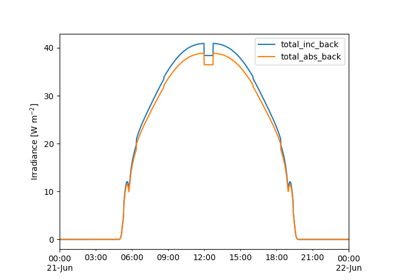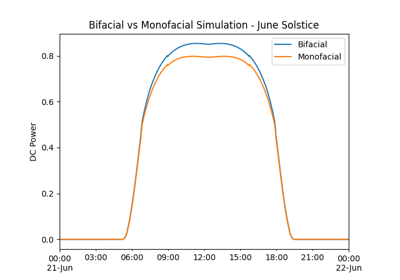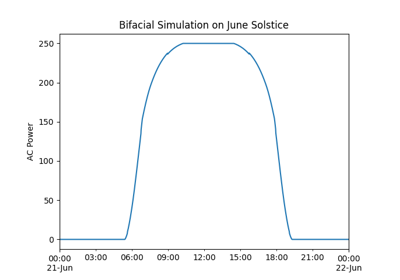# pvlib.bifacial.pvfactors.pvfactors_timeseries#

pvlib.bifacial.pvfactors.pvfactors_timeseries(solar_azimuth, solar_zenith, surface_azimuth, surface_tilt, axis_azimuth, timestamps, dni, dhi, gcr, pvrow_height, pvrow_width, albedo, n_pvrows=3, index_observed_pvrow=1, rho_front_pvrow=0.03, rho_back_pvrow=0.05, horizon_band_angle=15.0)[source]#

Calculate front and back surface plane-of-array irradiance on a fixed tilt or single-axis tracker PV array configuration, and using the open-source “pvfactors” package. pvfactors implements the model described in 1. Please refer to pvfactors online documentation for more details: https://sunpower.github.io/pvfactors/

Parameters
• solar_azimuth (numeric) – Sun’s azimuth angles using pvlib’s azimuth convention (deg)

• solar_zenith (numeric) – Sun’s zenith angles (deg)

• surface_azimuth (numeric) – Azimuth angle of the front surface of the PV modules, using pvlib’s convention (deg)

• surface_tilt (numeric) – Tilt angle of the PV modules, going from 0 to 180 (deg)

• axis_azimuth (float) – Azimuth angle of the rotation axis of the PV modules, using pvlib’s convention (deg). This is supposed to be fixed for all timestamps. When modeling fixed-tilt arrays, set this value to be 90 degrees clockwise from `surface_azimuth`.

• timestamps (datetime or DatetimeIndex) – List of simulation timestamps

• dni (numeric) – Direct normal irradiance (W/m2)

• dhi (numeric) – Diffuse horizontal irradiance (W/m2)

• gcr (float) – Ground coverage ratio of the pv array

• pvrow_height (float) – Height of the pv rows, measured at their center (m)

• pvrow_width (float) – Width of the pv rows in the considered 2D plane (m)

• albedo (float) – Ground albedo

• n_pvrows (int, default 3) – Number of PV rows to consider in the PV array

• index_observed_pvrow (int, default 1) – Index of the PV row whose incident irradiance will be returned. Indices of PV rows go from 0 to n_pvrows-1.

• rho_front_pvrow (float, default 0.03) – Front surface reflectivity of PV rows

• rho_back_pvrow (float, default 0.05) – Back surface reflectivity of PV rows

• horizon_band_angle (float, default 15) – Elevation angle of the sky dome’s diffuse horizon band (deg)

Returns

• poa_front (numeric) – Calculated incident irradiance on the front surface of the PV modules (W/m2)

• poa_back (numeric) – Calculated incident irradiance on the back surface of the PV modules (W/m2)

• poa_front_absorbed (numeric) – Calculated absorbed irradiance on the front surface of the PV modules (W/m2), after AOI losses

• poa_back_absorbed (numeric) – Calculated absorbed irradiance on the back surface of the PV modules (W/m2), after AOI losses

References

1

Anoma, Marc Abou, et al. “View Factor Model and Validation for Bifacial PV and Diffuse Shade on Single-Axis Trackers.” 44th IEEE Photovoltaic Specialist Conference. 2017.

## Examples using `pvlib.bifacial.pvfactors.pvfactors_timeseries`#Fixed-Tilt Simulation with pvfactors

Fixed-Tilt Simulation with pvfactorsBifacial Modeling - procedural

Bifacial Modeling - proceduralBifacial Modeling - modelchain

Bifacial Modeling - modelchain